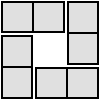#### You may also like### 4 Dom

Use these four dominoes to make a square that has the same number of dots on each side.### Just Rolling Round

P is a point on the circumference of a circle radius r which rolls, without slipping, inside a circle of radius 2r. What is the locus of P?### Areas and Ratios

Do you have enough information to work out the area of the shaded quadrilateral?

# Into the Wilderness

##### Age 14 to 18Challenge Level

This resource is part of our Adventures with Complex Numbers collection

This activity follows on from Strolling Along.

To multiply two complex numbers we can expand brackets, taking care to remember that $i^2 = -1$.

For example:

\eqalign{\left(4+2i\right)\left(\frac{7}{5}- 3i\right) &= \frac{28}{5} + \frac{14}{5}i - 12i - 6i^2 \\ &= \left(\frac{28}{5} + 6\right) + \left(\frac{14}{5} - 12\right)i \\ &= \frac{58}{5} - \frac{46}{5}i.}

But what's happening geometrically when we multiply complex numbers?

We have created the GeoGebra interactivity below for you to explore.
$z_3$ is the product $z_1z_2$.

You can move the complex number $z_1$ around the circle, and you can move $z_2$ anywhere on the grid. Use the slider $a$ to change the radius of the circle.

Choose a radius, and a position for $z_2$.
Move $z_1$ around the circle.

What do you notice?

Now explore what happens when you fix $z_1$ and move $z_2$.
You may find it helpful to "Show Lines" joining each point to the origin.

For which values of $z_2$ does the line through $z_1$ and $z_3$ pass through the origin?

What is special about the positions of $z_1$ for which $z_2$ and $z_3$ are equidistant from the origin?

Now that you have explored multiplication of numbers, you might like to try the Complex Puzzle.